Next: The scalar product Up: Vectors Previous: Vector algebra

## Vector areas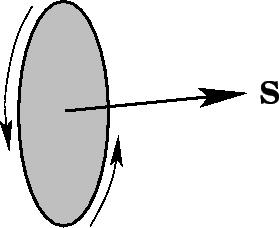Suppose that we have planar surface of scalar area. We can define a vector area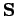whose magnitude is, and whose direction is perpendicular to the plane, in the sense determined by the right-hand grip rule on the rim (see Fig. 4). This quantity clearly possesses both magnitude and direction. But is it a true vector? We know that if the normal to the surface makes an angle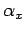with the-axis then the area seen looking along the-direction is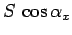. This is the-component of. Similarly, if the normal makes an angle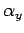with the-axis then the area seen looking along the-direction is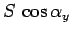. This is the-component of. If we limit ourselves to a surface whose normal is perpendicular to the-direction then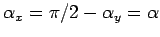. It follows that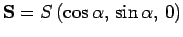. If we rotate the basis about the-axis bydegrees, which is equivalent to rotating the normal to the surface about the-axis by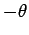degrees, then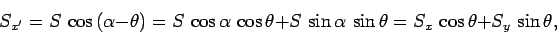(13)

which is the correct transformation rule for the-component of a vector. The other components transform correctly as well. This proves that a vector area is a true vector.

According to the vector addition theorem, the projected area of two plane surfaces, joined together at a line, looking along the-direction (say) is the-component of the resultant of the vector areas of the two surfaces. Likewise, for many joined-up plane areas, the projected area in the-direction, which is the same as the projected area of the rim in the-direction, is the-component of the resultant of all the vector areas: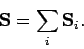(14)

If we approach a limit, by letting the number of plane facets increase, and their areas reduce, then we obtain a continuous surface denoted by the resultant vector area: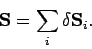(15)

It is clear that the projected area of the rim in the-direction is just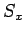. Note that the rim of the surface determines the vector area rather than the nature of the surface. So, two different surfaces sharing the same rim both possess the same vector area.

In conclusion, a loop (not all in one plane) has a vector areawhich is the resultant of the vector areas of any surface ending on the loop. The components ofare the projected areas of the loop in the directions of the basis vectors. As a corollary, a closed surface has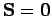, since it does not possess a rim.Next: The scalar product Up: Vectors Previous: Vector algebra
Richard Fitzpatrick 2006-02-02